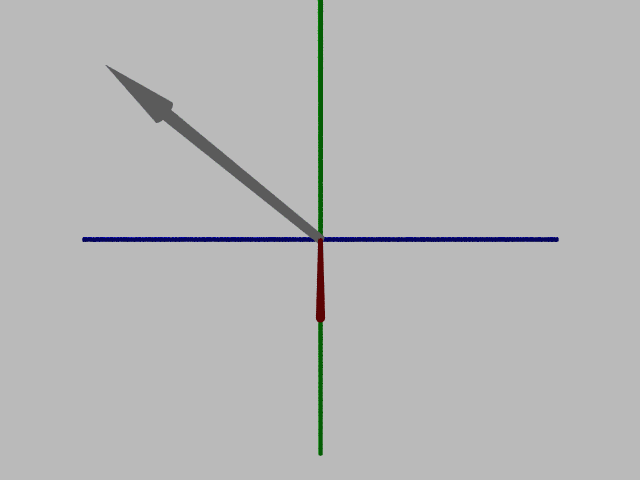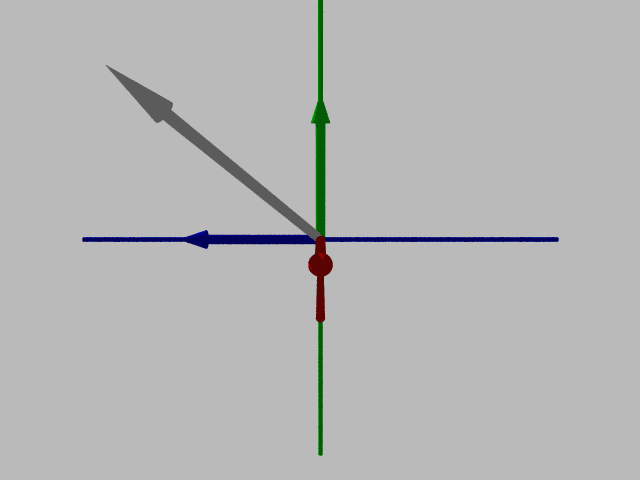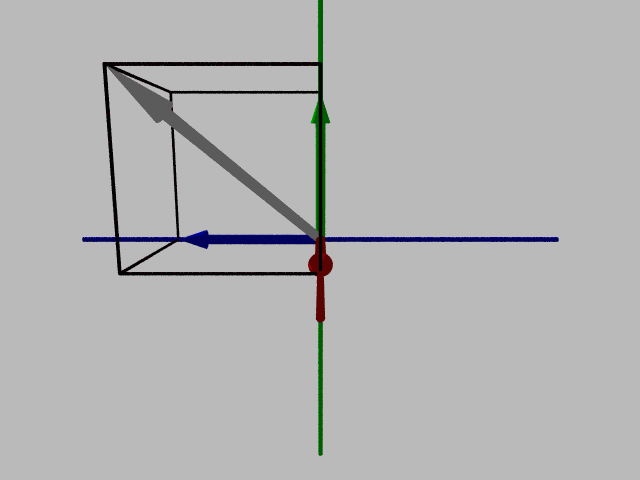# 3D Vector ComponentsHere is a quick jump to an interactive 3D virtual reality world that demonstrates the ideas presented below.

2D vectors have x- and y-components, as shown on these pages:

3D vectors have x-, y-, and z-components. First, let's look below at a 3D vector (white) in a three dimensional coordinate system:Shown below are the three components to this three dimensional vector:

• The x-component is along the x-axis (red)
• The y-component is along the y-axis (green)
• The z-component is along the z-axis (blue)Often, it is handy to see the 3D (white) vector and its three components enclosed in a box. This can help you see how all of these vectors line up and relate to each other: